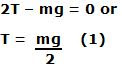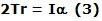## Wednesday, November 12, 2008

### Irodov Problem 1.244The arrangement is shown in the figure beside. The force of gravity pulls the Maxwell's disc downwards. The rod is accelerated upwards at a rate a such that, the Maxwell's disc remains in place and does not move downwards and the string unwinds.

Let the tension in each of the strings be T. There are three forces acting on the Maxwell's disc, i) the tensions from each of the string pulling the disc upwards and ii) the force of gravity mg. Since the Maxwell's disc does not move, its acceleration is 0. From Newton's laws we have,Let the acceleration of the rod be a and the angular acceleration of the Maxwell's disc as the thread unwinds be. Clearly, these two quatities are related as,since the thread does not slip.

The tension in the strings induces a torque on the Maxwell's disc equal to 2Tr. Given that the moment of inertia of the Maxwell's disc is I, we have,From (1), (2) and (3) we have,